# Similar Triangles

Similar triangles are triangles that have the same shape, but their sizes may vary. All equilateral triangles, squares of any side lengths are examples of similar objects. In other words, if two triangles are similar, then their corresponding angles are congruent and corresponding sides are in equal proportion. We denote the similarity of triangles here by ‘~’ symbol.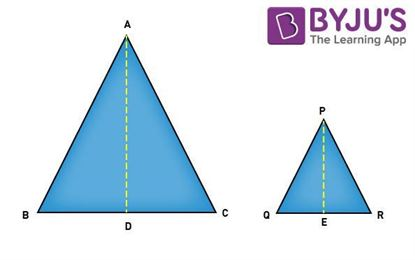## Definition

Two triangles are similar if they have the same ratio of corresponding sides and equal pair of corresponding angles.

If two or more figures have the same shape, but their sizes are different, then such objects are called similar figures. Consider a hula hoop and wheel of a cycle, the shapes of both these objects are similar to each other as their shapes are the same.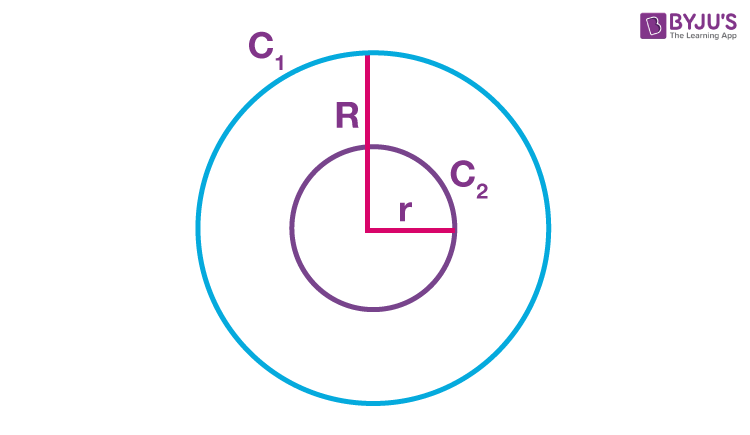In the figure given above, two circles C1 and C2 with radius R and r respectively are similar as they have the same shape, but necessarily not the same size. Thus, we can say that C1~ C2.

It is to be noted that, two circles always have the same shape, irrespective of their diameter. Thus, two circles are always similar.

Triangle is the three-sided polygon. The condition for the similarity of triangles is;

i)        Corresponding angles of both the triangles are equal, and
ii)       Corresponding sides of both the triangles are in proportion to each other.

### Similar Triangle Example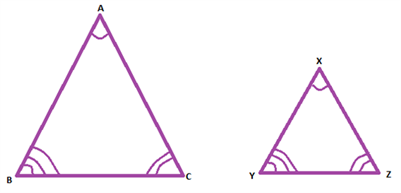In the given figure, two triangles ΔABC and ΔXYZ are similar only if,

i)        ∠A = ∠X, ∠B = ∠Y and ∠C = ∠Z
ii)       AB/XY = BC/YZ = AC/XZ (Similar triangles proportions)

Hence, if the above-mentioned conditions are satisfied, then we can say that ΔABC ~ ΔXYZ

It is interesting to know that if the corresponding angles of two triangles are equal, then such triangles are known as equiangular triangles. For two equiangular triangles we can state the Basic Proportionality Theorem (better known as Thales Theorem) as follows:

• For two equiangular triangles, the ratio of any two corresponding sides is always the same.

## Properties

• Both have the same shape but sizes may be different
• Each pair of corresponding angles are equal
• The ratio of corresponding sides is the same

## Formulas

According to the definition, two triangles are similar if their corresponding angles are congruent and corresponding sides are proportional. Hence, we can find the dimensions of one triangle with the help of another triangle. If ABC and XYZ are two similar triangles, then by the help of below-given formulas, we can find the relevant angles and side lengths.

• ∠A = ∠X, ∠B = ∠Y and ∠C = ∠Z
• AB/XY = BC/YZ = AC/XZ

Once we have known all the dimensions and angles of triangles, it is easy to find the area of similar triangles.

## Similar Triangles and Congruent Triangles

The comparison of similar triangles and congruent triangles is given below in the table.

 Similar Triangles Congruent Triangles They are the same shape but different in size They are the same in shape and size Symbol is ‘~’ Symbol is ‘≅’ Ratio of all the corresponding sides are same Ratio of corresponding sides are equal to a constant value

#### To Know how to Find the Area Of Similar Triangles, Watch The Below Video:## Similar triangles Theorems with Proofs

Let us learn here the theorems used to solve the problems based on similar triangles along with the proofs for each.

### AA (or AAA) or Angle-Angle Similarity

If any two angles of a triangle are equal to any two angles of another triangle, then the two triangles are similar to each other.

From the figure given above, if ∠ A = ∠X and ∠C = ∠Z then ΔABC ~ΔXYZ.

From the result obtained, we can easily say that,

AB/XY = BC/YZ = AC/XZ

and ∠B = ∠Y

### SAS or Side-Angle-Side Similarity

If the two sides of a triangle are in the same proportion of the two sides of another triangle, and the angle inscribed by the two sides in both the triangle are equal, then two triangles are said to be similar.

Thus, if ∠A = ∠X and AB/XY = AC/XZ then ΔABC ~ΔXYZ.

From the congruency,

AB/XY = BC/YZ = AC/XZ

and ∠B = ∠Y and ∠C = ∠Z

### SSS or Side-Side-Side Similarity

If all the three sides of a triangle are in proportion to the three sides of another triangle, then the two triangles are similar.

Thus, if AB/XY = BC/YZ = AC/XZ then ΔABC ~ΔXYZ.

From this result, we can infer that-

∠A = ∠X, ∠B = ∠Y and ∠C = ∠Z

## Problem and Solutions

Let us go through an example to understand it better.
Q.1: In theΔABC length of the sides are given as AP = 5 cm , PB = 10 cm and BC = 20 cm. Also PQ||BC. Find PQ.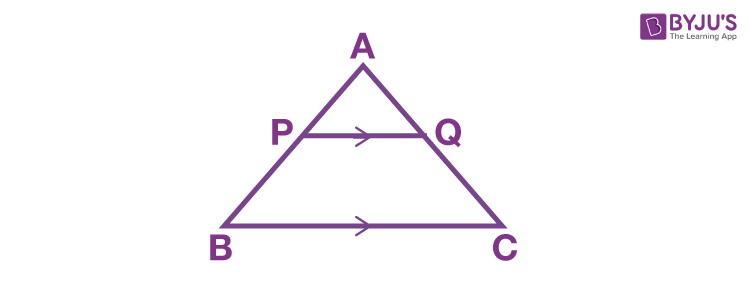Solution: In ΔABC and ΔAPQ, ∠PAQ is common and ∠APQ = ∠ABC (corresponding angles)

⇒ ΔABC ~ ΔAPQ (AA criterion for similar triangles)

AP/AB = PQ/BC

⇒ 5/15 = PQ/20

⇒ PQ = 20/3 cm

Q.2: Diagonals AC and BD of a trapezium ABCD with AB || DC intersect each other at the point O. Using a similarity criterion for two triangles, show that AO/OC = OB/OD.

Solution: ABCD is a trapezium and O is the intersection of diagonals AC and BD.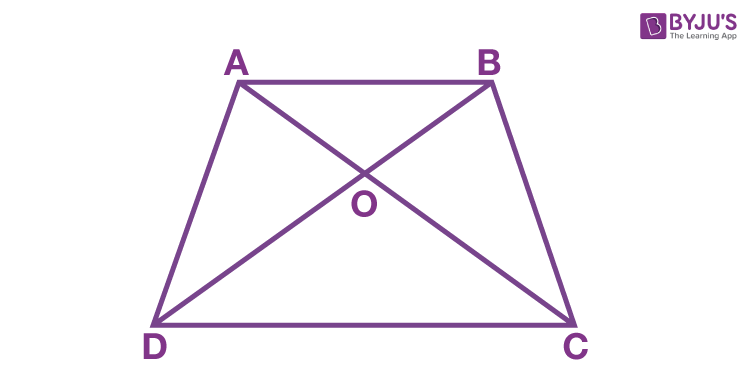In ΔDOC and ΔBOA,

AB || CD, thus alternate interior angles will be equal,

∴∠CDO = ∠ABO

Similarly,

∠DCO = ∠BAO

Also, for the two triangles ΔDOC and ΔBOA, vertically opposite angles will be equal;

∴∠DOC = ∠BOA

Hence, by AAA similarity criterion,

ΔDOC ~ ΔBOA

Thus, the corresponding sides are proportional.

DO/BO = OC/OA

⇒OA/OC = OB/OD

Hence, proved.

Q.3: Check if the two triangles are similar.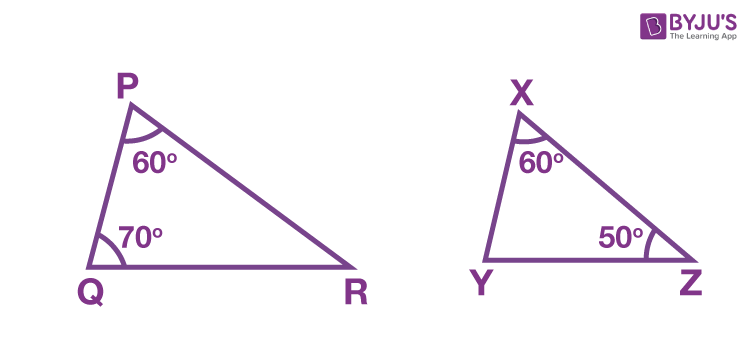Solution: In triangle PQR, by angle sum property;

∠P + ∠Q + ∠R = 180°

60° + 70° + ∠R = 180°

130° + ∠R = 180°

Subtract both sides by 130°.

∠ R= 50°

Again in triangle XYZ, by angle sum property;

∠X + ∠Y + ∠Z = 180°

∠60° + ∠Y + ∠50°= 180°

∠ 110° + ∠Y = 180 °

Subtract both sides by 110°

∠ Y = 70°

Since,∠Q = ∠ Y = 70° and ∠Z = ∠ R= 50°

Therefore, by Angle-Angle (AA) rule,

ΔPQR~ΔXYZ.

## Similar Triangles Video LessonThis video will help you visualize basic criteria for the similarity of triangles.

## Frequently Asked Questions – FAQs

Q1

### What are similar triangles?

Two triangles are similar if they have the same ratio of corresponding sides and equal pair of corresponding angles.
Q2

### What is the symbol for similar triangles?

If ABC and PQR are two similar triangles, then they are represented by:
∆ABC ~ ∆PQR
Q3

### Are similar triangles and congruent triangles same?

Similar triangles have the same shape but sizes may vary but congruent triangles have the same shape and size. Congruent triangles are represented by symbol ‘≅’.
Q4

### What are three similarities theorems for triangles?

The three similarities theorem are:
Angle-angle (AA)
Side-angle-side (SAS)
Side-side-side (SSS)
Q5

### How to find the proportion of similar triangles?

If two triangles are similar and have sides A,B,C and a,b,c, respectively, then the pair of corresponding sides are proportional, i.e.,
A : a = B : b = C : c.